# 基于粒子群算法的深度置信网络焙烧过程可溶锌率预测Prediction of Soluble Zinc Rate during Roasting Process Based on Particle Swarm Optimization in Deep Belief Network

• 全文下载: PDF(1423KB)    PP.141-153   DOI: 10.12677/CSA.2020.101016
• 下载量: 73  浏览量: 112   国家自然科学基金支持

In order to solve the problem that the soluble zinc ratio is difficult to be measured online in the roasting quality of zinc smelting and roasting process, a Deep Belief Network (DBN) algorithm was proposed to predict the soluble zinc rate. However, the DBN network structure is an important factor affecting its prediction performance, and it is difficult to determine the appropriate network structure. It is proposed to use the information entropy method to determine the appropriate number of hidden layer, then use the PSO algorithm to optimize the number of hidden layer nodes and learning rate, and finally determine the DBN network structure. The method was validated by data set simulation and practical application of soluble zinc rate prediction, and compared with BP neural network and RBF neural network models. The results show that the DBN network structure optimized by the information entropy method and the PSO algorithm has higher prediction accuracy and stronger fitting ability.

1. 引言

DBN网络的特点是通过深层次的网络结构，逐层学习训练的方式提高对复杂非线性函数拟合能力，进而提高模型的性能。因此要确定一个合适的网络结构。在实际的应用中，没有成熟的理论帮助确定网络结构，一般是依据积累的经验，多次实验去选择合适的网络结构。Shen  通过人工反复试验确定的DBN网络结构，但其确定的网络结构复杂且不合理，造成DBN模型运行时间长，模型性能较差。因此，如何优化出最适合的网络结构，对DBN在人工智能领域的应用研究，具有重要的意义。针对这个问题，首先通过信息熵法确定隐层层数，然后采用PSO算法对DBN网络结构的神经元个数和学习率进行优化，确定一个合适的DBN网路结构。这样能够避免模型结构参数选择的盲目性，减少其对模型精度的影响。

2. DBN预测模型

DBN是根据生物神经网络的研究及浅层神经网络发展而来的，为概率生成模型，通过联合概率分布推断出数据样本分析。DBN生成模型通过训练网络结构中的神经元间的权重使得整个神经元网络依据最大概率生成训练数据，形成高层抽象特征，提升模型性能。

2.1. 深度置信网络

RBM由两层组成，如图1所示。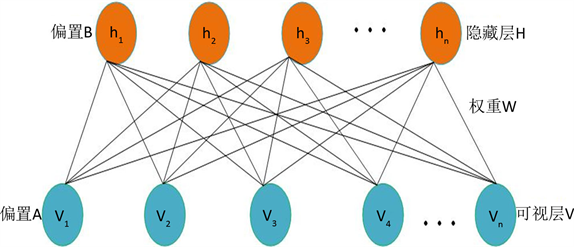Figure 1. RBM network structure

$E\left(v,h|\theta \right)=-{\sum }_{i=1}^{m}{\sum }_{j=1}^{n}{v}_{i}{w}_{ij}{h}_{j}-{\sum }_{i=1}^{m}{a}_{i}{v}_{i}-{\sum }_{j}^{n}{b}_{j}{h}_{j}$ (2.1)

$P\left(v,h|\theta \right)=\frac{{\text{e}}^{-E\left(v,h|\theta \right)}}{Z\left(\theta \right)}$ (2.2)

$Z\left(\theta \right)={\sum }_{v,h}{\text{e}}^{-E\left(v,h|\theta \right)}$ (2.3)

$P\left(v|\theta \right)=\frac{{\sum }_{h}{\text{e}}^{-E\left(v,h|\theta \right)}}{{Z}^{\theta }}$ (2.4)

$P\left(h|v,\theta \right)={\prod }_{i}P\left({v}_{i}|h\right)$ (2.5)

$P\left(h|v,\theta \right)={\prod }_{i}P\left({h}_{i}|v\right)$ (2.6)

$P\left({v}_{i}=1|h,\theta \right)=\sigma \left({a}_{i}+{\sum }_{j}{h}_{i}{\omega }_{ij}\right)$ (2.7)

$P\left({h}_{i}=1|v,\theta \right)=\sigma \left({b}_{i}+{\sum }_{j}{v}_{i}{\omega }_{ij}\right)$ (2.8)

DBN使用无监督贪婪算法自下而上的逐层训练学习产生各层之间的权值。训练过程中，首先可视层单元映射到隐藏层单元，隐藏层单元再反射过来重构可视层单元，反复执行这个步骤得到权值。每一层RBM隐藏层的输出作为下一层RBM可视层的输入。与传统神经网络的算法不同的是，DBN的训练只需要单个步骤就能实现最大程度的函数逼近，训练时间明显减少。在上面的预训练过程结束后，DBN使用有标签数据通过BP算法有监督的反向传播来微调预训练生成的权值，得到较为精确的预测模型。DBN网络结构如图2所示。Figure 2. Structure of DBN network prediction model

2.2. 数据集选择及评价指标

$\text{MSE}=\frac{1}{n}{\sum }_{i=1}^{n}{\left({y}_{i}-{\stackrel{^}{y}}_{i}\right)}^{2}$ (2.9)

${\text{R}}^{2}=\frac{{\sum }_{i=1}^{n}{\left({\stackrel{^}{y}}_{i}-\stackrel{¯}{y}\right)}^{2}}{{\sum }_{i=1}^{n}{\left({y}_{i}-\stackrel{¯}{y}\right)}^{2}}$ (2.10)

$\text{MAPE}=\frac{1}{n}{\sum }_{i=1}^{n}|\frac{{y}_{i}-{\stackrel{^}{y}}_{i}}{{y}_{i}}|$ (2.11)

2.3. 数据预处理

${X}^{\prime }=\frac{X-{X}_{\mathrm{min}}}{{X}_{\mathrm{max}}-{X}_{\mathrm{min}}}$ (2.12)

3. 基于信息熵和PSO的DBN结构优化

3.1. DBN网络结构对性能的影响

DBN网络结构设计就是选择合适的隐层层数、神经元个数及网络参数来确定网络结构。在DBN网络中，如果隐层层数选择得当，模型性能可以明显的提高。通过增加隐层层数不仅可以降低模型的重构误差，而且能挖掘出数据中更为抽象的特征信息，由于隐层层数的增加网络结构通常会更加复杂，若隐层层数过少，模型的学习能力不足，不能较好的解决实际问题。因此，要选取合适的隐层层数。

3.2. 基于信息熵确定DBN网络隐层层数

$H\left(x\right)=E\left\{\mathrm{log}\frac{1}{p\left({x}_{i}\right)}\right\}=-{\sum }_{i=1}^{n}P\left({x}_{i}\right){\mathrm{log}}_{2}\left(P\left({x}_{i}\right)\right)$ (3.1)

$G\left(P\left({x}_{1}\right),P\left({\chi }_{2}\right),\cdots ,P\left({x}_{n}\right),\lambda \right)=-{\sum }_{i=1}^{n}P\left({x}_{i}\right){\mathrm{log}}_{2}\left(P\left({x}_{i}\right)\right)+\lambda \left({\sum }_{i=1}^{n}p\left({x}_{i}\right)\right)-1$ (3.2)

$\frac{\alpha G}{\alpha P\left({x}_{i}\right)}=0$$\frac{\alpha G}{\lambda }=0$，可以得到：

$\frac{\alpha G}{\alpha P\left({x}_{i}\right)}=-{\mathrm{log}}_{2}p\left({x}_{i}\right)-1+\lambda =0;\text{\hspace{0.17em}}i=1,2,3,\cdots ,n$ (3.3)

${\sum }_{i=1}^{n}P\left({x}_{i}\right)-1=0$ (3.4)

$p\left({x}_{i}\right)=\mathrm{exp}\left(\lambda -1\right),\text{\hspace{0.17em}}i=1,2,3,\cdots ,n$ (3.5)

$P\left({x}_{1}\right)=P\left({\chi }_{2}\right)=\cdots =P\left({x}_{n}\right)=\frac{1}{n}$ (3.6)

$P\left({x}_{1}\right)=P\left({\chi }_{2}\right)=\cdots =P\left({x}_{n}\right)=\frac{1}{n}$ 代入式(19)得：

${H}_{\mathrm{max}}\left(n\right)=\mathrm{log}{2}^{\left(n\right)}=H\left(1/n,2/n,\cdots ,1/n\right)\ge H\left({p}_{1},{p}_{2},\cdots ,{p}_{n}\right)$ (3.7)

$W=\left[\begin{array}{ccc}{W}_{11}& \cdots & {W}_{n1}\\ ⋮& \ddots & ⋮\\ {W}_{1m}& \cdots & {W}_{nm}\end{array}\right]$

${h}_{j}={b}_{j}+{v}_{i}\ast {w}_{ij}$ (3.8)

$\eta =\frac{m}{n}$ (3.9)

$\eta =\frac{H隐层}{H输入层}$ (3.10)

$n=m\frac{H隐层}{H输入层}$ (3.11)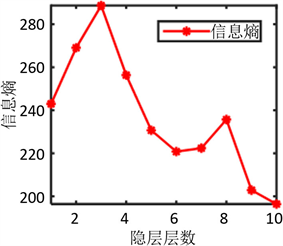Figure 3. Information entropy of different hidden layer NumbersTable 1. Performance prediction of DBN model under different hidden layers

3.3. 基于PSO-DBN网络结构优化方法

3.3.1. 粒子群算法

PSO算法中，搜索空间上的每一个点都可以被想象成待优化问题的潜在解，这个点称之为“粒子”(Particle)，任何粒子都有一个被目标函数所决定的适应值，并且粒子移动的方向和距离都会有一个速度决定，然后粒子们就追随当前最优粒子在解空间搜索。其中粒子的速度和运动方向更新公式如下：

$\begin{array}{c}v\left[i+1\right]=w×v\left[i\right]+{c}_{1}×rand\left(\text{ }\right)×\left(pbest\left[i\right]-present\left[i\right]\right)\\ \text{\hspace{0.17em}}\text{\hspace{0.17em}}+{c}_{2}×rand\left(\text{ }\right)×\left(gbest-present\left[i\right]\right)\end{array}$ (3.12)

$present\left[i+1\right]=present\left[i\right]+v\left[i+1\right]$ (3.13)

3.3.2. PSO-DBN模型训练过程

PSO算法流程：

Input：种群规模m，惯性权重w，加速常数c1，c2，最大速度Vmax，最大迭代次数Gmax

Output：DBN网络待优化参数。

1：初始化一群粒子 $Pop{\left\{i\right\}}_{i=1}^{m}$ (规模为m)，随机初始化它们的位置和速度；

2：根据目标函数，计算每个微粒的适应度值；

3：训练DBN算法；

4：对每个粒子，将它当前的适应度值和它经历过的最好位置(pbest)的值比较，若当前较好，则将其作为新的最好位置pbest

5：对每个粒子，将它当前的适应度值和它整个种群的最好位置(gbest)的值比较，若当前较好，则将其作为新的种群最好位置gbest

6：按照方程(9)和(10)更新每个粒子的速度和位置；

7：判断是否达到结束条件(通常为足够好的适应值或达到最大迭代次数Gmax)，不满足就返回2，若满足则输出为DBN网络模型参数。

DBN网络训练两个阶段是预训练和反向微调。

Step1：模型预训练：

Step2：权值微调：

$f\left(\theta \right)=-{\sum }_{i}{y}_{i}^{\text{T}}\mathrm{log}{{y}^{\prime }}_{i}$ (3.14)

PSO-DBN训练流程如图4所示。

3.4. 仿真验证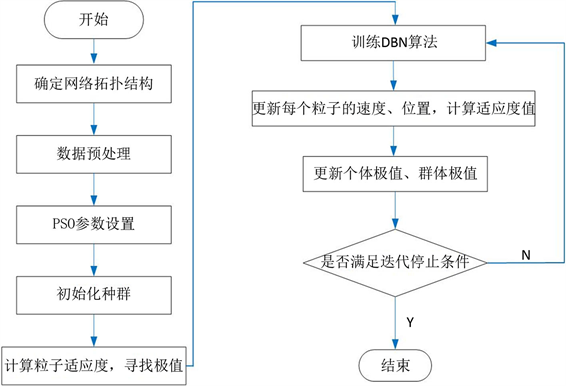Figure 4. PSO-DBN network prediction model training process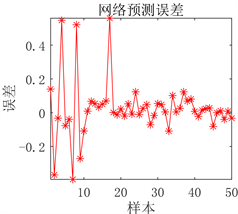(a) 预测图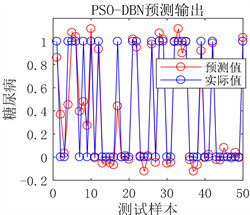(b) 预测误差图

Figure 5. Prediction results of DBN network prediction model before optimization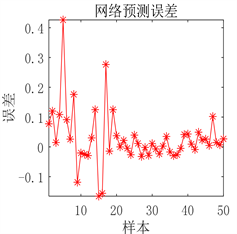(a) 预测图(b) 预测误差图

Figure 6. Prediction results of DBN network prediction model after optimizationTable 2. Experimental parameters and PSO optimization network parameters

4. 应用研究Table 3. Parameter setting of PSO algorithmTable 4. Comparison of experimental parameters and PSO optimization network parameters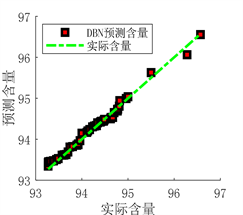(a) 预测图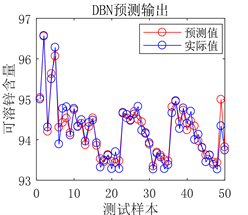(b) 实际值与预测值拟合图

Figure 7. Prediction results of DBN network prediction model before optimization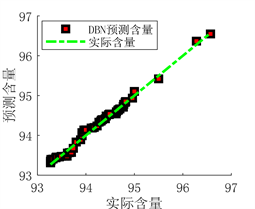(a) 预测图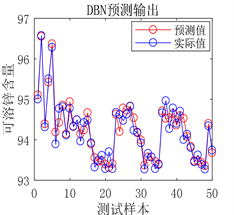(b) 实际值与预测值拟合图

Figure 8. Prediction results of DBN network prediction model after optimization

DBN模型参数优化前后评价结果量化如表5所示：Table 5. Comparison of DBN model experimental parameters and PSO optimization parameters prediction and evaluation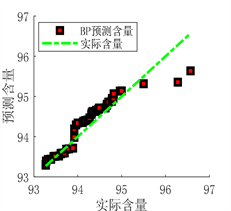(a) 预测图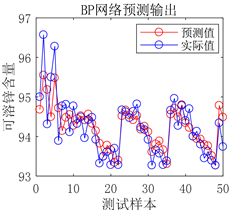(b) 实际值与预测值拟合图

Figure 9. Prediction results of BP neural network prediction model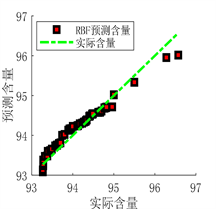(a) 预测图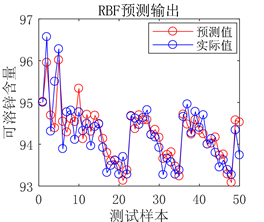(b) 实际值与预测值拟合图

Figure 10. Prediction results of RBF neural network prediction modelTable 6. Comparison of performance indexes of soluble zinc rate prediction model

5. 结束语

  Lee, T.S. and Mumford, D. (2003) Hierarchical Bayesian Inference in the Visual Cortex. Journal of the Optical Society of America, 20, 1434-1448.  潘广源, 柴伟, 乔俊飞. DBN网络的深度确定方法[J]. 控制与决策, 2015, 30(2): 256-260.  Hinton, G.E. and Salakhutdinov, R.R. (2006) Reducing the Dimensionality of Data with Neural Net-works. Science, 313, 504-507. https://doi.org/10.1126/science.1127647  Dahl, G.E., Yu, D., Deng, L. and Ac-ero, A. (2011) Large Vocabulary Continuous Speech Recognition with Context-Dependent DBN-HMMS. Proceedings of the IEEE International Conference on Acoustics, Speech, and Signal Processing, ICASSP 2011, Prague Congress Center, Prague, 22-27 May 2011, 4688-4691. https://doi.org/10.1109/ICASSP.2011.5947401  徐春华, 陈克绪, 马建, 刘佳翰, 吴建华. 基于深度置信网络的电力负荷识别[J]. 电工技术学报, 2019, 34(19): 4135-4142.  毛勇华, 代兆胜, 桂小林. 一种改进的5层深度学习结构与优化方法[J]. 计算机工程, 2018, 44(6): 147-150.  Wang, Y.B., You, Z.H., Li, X., et al. (2017) Predicting Protein-Protein Interactions from Protein Sequences by a Stacked Sparse Autoencoder Deep Neural Network. Molecular Biosystems, 13, 1336-1344. https://doi.org/10.1039/C7MB00188F  Liu, F., Jiao, L.C., Hou, B. and Yang, S.Y. (2016) POL-SAR Image Classification Based on Wishart DBN and Local Spatial Information. IEEE Transactions on Geoscience & Remote Sens-ing, 54, 1-17. https://doi.org/10.1109/TGRS.2016.2514504  Kong, W., Zhao, Y.D., Hill, D.J., Luo, F. and Xu, Y. (2018) Short-Term Residential Load Forecasting Based on Resident Behaviour Learning. IEEE Transactions on Power Systems, 33, 1087-1088. https://doi.org/10.1109/TPWRS.2017.2688178  Li, B.-Q., He, Y.-Y., Guo, Y.-S. and Qiu, Y. (2017) Auto-matic Interpretation Algorithm for Tunnel Geological Prediction Based on DBN. Journal of Chang’an University (Natu-ral Science Edition), 37, 90-96.  高月, 宿翀, 李宏光. 一类基于非线性PCA和深度置信网络的混合分类器及其在PM2.5浓度预测和影响因素诊断中的应用[J]. 自动化学报, 2018, 44(2): 318-329.  Zhou, S.Z.S., Chen, Q.C.Q. and Wang, X.W.X. (2010) Discriminative Deep Belief Networks for Image Classification. 2010 IEEE Interna-tional Conference on Image Processing, Hong Kong, 26-29 September 2010, 1561-1564. https://doi.org/10.1109/ICIP.2010.5649922  张媛媛, 霍静, 杨婉琪, 等. 深度信念网络的二代身份证异构人脸核实算法[J]. 智能系统学报, 2015, 10(2): 193-200.  朱乔木, 党杰, 陈金富, 徐友平, 李银红, 段献忠. 基于深度置信网络的电力系统暂态稳定评估方法[J]. 中国电机工程学报, 2018, 38(3): 735-743.  张楠, 丁世飞, 张健, 赵星宇. 基于噪声数据与干净数据的深度置信网络[J]. 软件学报, 2019, 30(11): 3326-3339.  Lv, Y., Duan, Y., Kang, W., Li, Z. and Wang, F.-Y. (2015) Traffic Flow Prediction With Big Data: A Deep Learning Ap-proach. IEEE Transactions on Intelligent Transportation Systems, 16, 865-873.  许冬. 复杂锌精矿沸腾焙烧预测神经网络研究[D]: [硕士学位论文]. 西安: 西安建筑科技大学, 2008.  Shen, F., Chao, J. and Zhao, J. (2015) Forecasting Exchange Rate Using Deep Belief Networks and Conjugate Gradient Method. Neurocomputing, 167, 243-253. https://doi.org/10.1016/j.neucom.2015.04.071  张国辉. 基于深度置信网络的时间序列预测方法及其应用研究[D]: [硕士学位论文]. 哈尔滨: 哈尔滨工业大学, 2017.